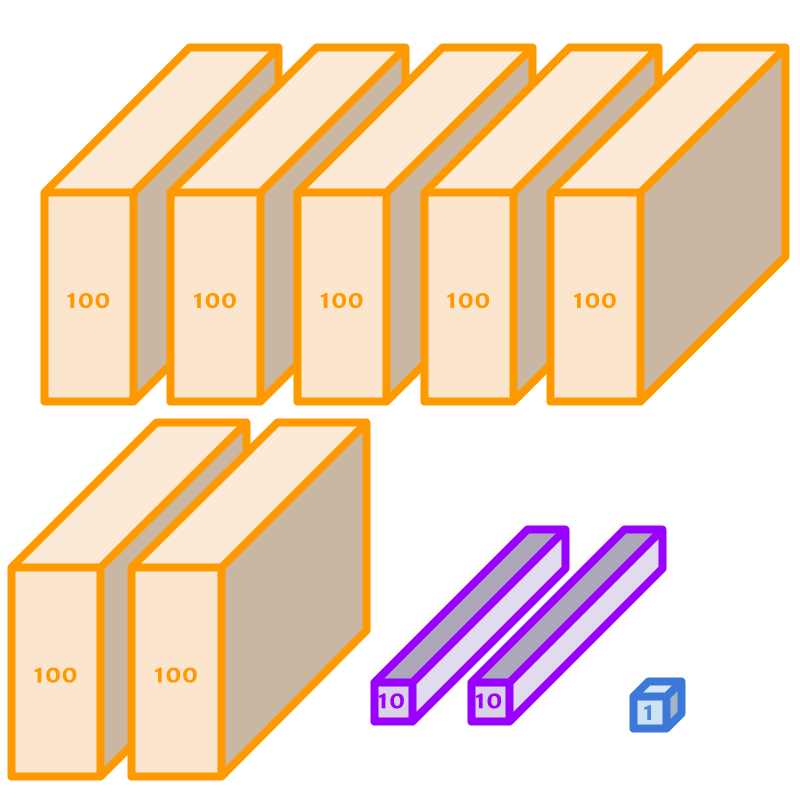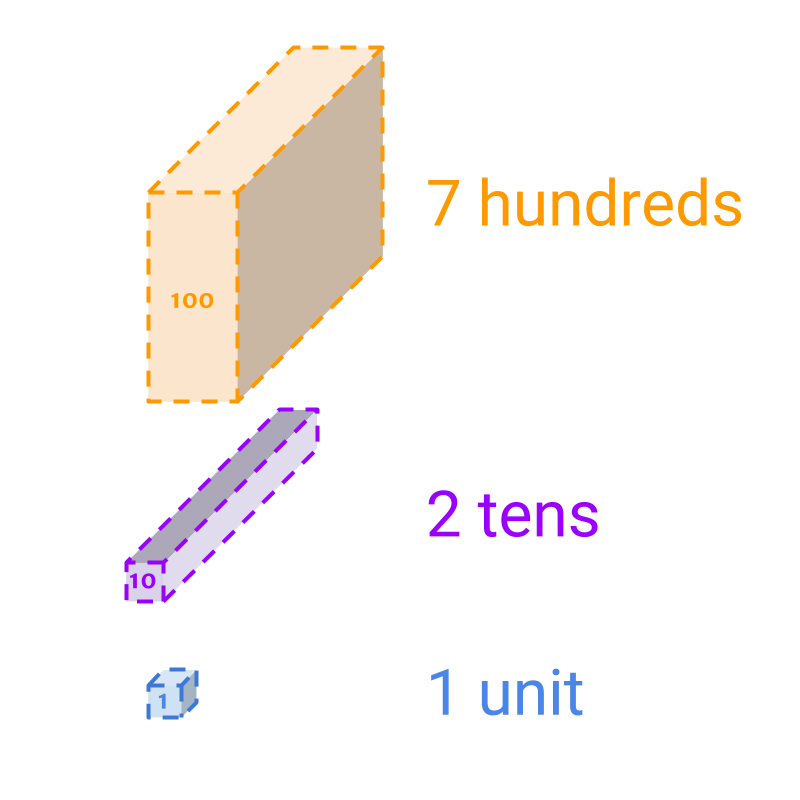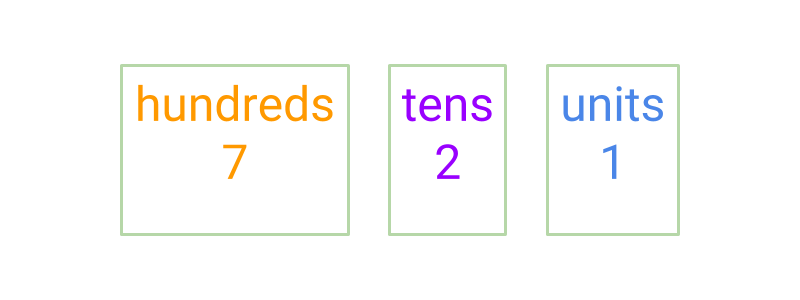maths > wholenumbers

Face and Place Values

what you'll learn...

overview

This page formalizes the value of the digits in a number as follows.

•  face value : the count represented by the digit.
eg: 2$2$ has face value of 2$2$ in 235$235$.

•  place value : the value represented by the digit as part of the number.
eg: 2$2$ has place value of 200$200$ in 235$235$.

Face and Place

We learned about representation of the number $721$ in three forms. Let us revise that quickly.The number $721$ is represented using blocks.The number is represented using the count of each of the blocks. Instead of laying out all the blocks, the representation gives the count of each of the blocks. The blocks are of sizes $1$, $10$, and $100$.The number is represented without blocks. Instead of providing a pictorial representation of the blocks, the value is given in letters. The values are hundreds, tens, and units, equivalently representing blocks of size $100$, $10$, and $1$.

The word "value" means: amount; measured amount; counted number.

The word "face", in face-value means: value represented by the symbol, without any context or details. That is, something is presented and taken as it is, without analyzing.

The word "place" in place-value means: a specific position or location.

Face Value: For a digit in a number, the face value is the value of the digit. Eg: $2$ has face value of $2$ in $235$.

Place Value: For a digit in a number, the place value is the value taken by the digit as part of the number. Eg: $2$ has place value of $200$ in $235$.

Some examples

The face value of $2$ in the number $5234$ is $2$.

The place value of $2$ in the number $5234$ is $200$.

The face value of $3$ in $2341$ is $3$.

The place value of $2$ in $6234$ is $200$.

The face value of $6$ in $16425$ is $6$.

Summary

Face Value: For a digit in a number, the face value is the value of the digit. Eg: $2$ has face value of $2$ in $235$.

Place Value: For a digit in a number, the place value is the value taken by the digit as part of the number. Eg: $2$ has place value of $200$ in $235$.

Outline